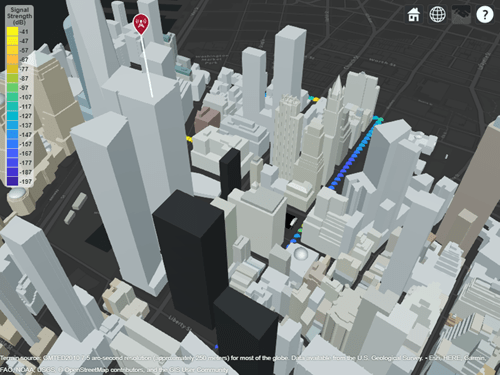# propagationData

Create RF propagation data from measurements

## Description

Use the `propagationData` object to import and visualize geolocated propagation data. The measurement data can be path loss data, signal strength measurements, signal-to-noise-ratio (SNR) data, or cellular information.

## Creation

### Syntax

``pd = propagationData(filename)``
``pd = propagationData(table)``
``pd = propagationData(latitude,longitude,varname,varvalue)``
``pd = propagationData(___,Name,Value)``

### Description

````pd = propagationData(filename)` creates a propagation data object by reading data from a file specified by `filename`.```
````pd = propagationData(table)` creates a propagation data container object from a table object specified by `table`.```

example

````pd = propagationData(latitude,longitude,varname,varvalue)` creates a propagation data container object using `latitude` and `longitude` coordinates with data specified using `varname` and `varvalue`.```

example

````pd = propagationData(___,Name,Value)` sets properties using one or more name-value pairs. Enclose each property name in quotes.```

### Input Arguments

expand all

Name of the file containing propagation data, specified as a character vector or a string scalar. The file must be in the current directory, in a directory on the MATLAB path, or be specified using a full or relative path. The file must be compatible with the `readtable` function. Call the `readtable` function if customized parameters are required to import the file and then pass the `table` object to the `propagationData` object.

Propagation data in the file must have one variable corresponding to the latitude values, one variable corresponding to longitude values, and at least one variable containing numeric data.

Data Types: `string` | `char`

Table containing propagation data, specified as a table object.

Propagation data in the file must have one variable corresponding to the latitude values, one variable corresponding to longitude values, and at least one variable containing numeric data.

Data Types: `table`

Latitude coordinate values, specified as a vector in decimal degrees with reference to Earth's ellipsoid model WGS-84. The latitude coordinates must be in the range `[-90 90]`.

Data Types: `double`

Longitude coordinate values, specified as a vector in decimal degrees with reference to earth's ellipsoid. model WGS-84.

Data Types: `double`

Variable name, specified as a character vector or a string scalar. This variable name must correspond to the variable with numeric data other than latitude or longitude. The variable name and the corresponding values are stored as a column in the Data property table object.

Data Types: `string` | `char`

Variable values, specified as a numeric vector. The numeric vectors must be the same size as latitude and longitude. The variable name and corresponding values are stored as a column in the Data property table object.

Data Types: `double`

### Output Arguments

expand all

Propagation data, returned as a `propagationData` object.

## Properties

expand all

Propagation data name, specified as a character vector or string scalar.

Example: `'Name','propdata'`

Example: `pd.Name = 'propdata'`

Data Types: `char` | `string`

Propagation data table, specified as a scalar table object containing a column corresponding to latitude coordinates, a column corresponding to longitude coordinates, and one or more columns corresponding to associated propagation data.

Data Types: `table`

Name of the data variable to plot, specified as a character vector or string scalar corresponding to a variable name in the `Data` table used to create propagation data container object. The variable name must correspond to a variable with numeric data and cannot correspond to the latitude or longitude variables. The default value for this property is the name of the first numeric data variable name in the `Data` table that is not a latitude or longitude variable.

Data Types: `char` | `string`

## Object Functions

 `plot` Display RF propagation data in Site Viewer `contour` Display contour map of RF propagation data in Site Viewer `location` Coordinates of RF propagation data `getDataVariable` Get data variable values `interp` Interpolate RF propagation data

## Examples

collapse all

Launch Site Viewer with basemaps and building files for Manhattan. For more information about the osm file, see .

```viewer = siteviewer("Basemap","streets_dark",... "Buildings","manhattan.osm");```Show a transmitter site on a building.

```tx = txsite("Latitude",40.7107,... "Longitude",-74.0114,... "AntennaHeight",80); show(tx)```Create receiver sites along nearby streets.

```latitude = [linspace(40.7088, 40.71416, 50), ... linspace(40.71416, 40.715505, 25), ... linspace(40.715505, 40.7133, 25), ... linspace(40.7133, 40.7143, 25)]'; longitude = [linspace(-74.0108, -74.00627, 50), ... linspace(-74.00627 ,-74.0092, 25), ... linspace(-74.0092, -74.0110, 25), ... linspace(-74.0110, -74.0132, 25)]'; rxs = rxsite("Latitude", latitude, "Longitude", longitude);```

Compute signal strength at each receiver location.

`signalStrength = sigstrength(rxs, tx)';`

Create a `propagationData` object to hold computed signal strength data.

```tbl = table(latitude, longitude, signalStrength); pd = propagationData(tbl);```

Plot the signal strength data on a map as colored points.

```legendTitle = "Signal" + newline + "Strength" + newline + "(dB)"; plot(pd, "LegendTitle", legendTitle, "Colormap", parula);```Appendix

Define names and locations of sites around Boston.

```names = ["Fenway Park","Faneuil Hall","Bunker Hill Monument"]; lats = [42.3467,42.3598,42.3763]; lons = [-71.0972,-71.0545,-71.0611];```

Create an array of transmitter sites.

```txs = txsite("Name",names,... "Latitude",lats,... "Longitude",lons, ... "TransmitterFrequency",2.5e9); show(txs) ```Create a signal-to-interference-plus-noise-ratio (SINR) map, where signal source for each location is selected as the transmitter site with the strongest signal.

```sv1 = siteviewer("Name","SINR map"); sinr(txs,"MaxRange",5000)```Return SINR propagation data.

```pd = sinr(txs,"MaxRange",5000); [sinrDb,lats,lons] = getDataVariable(pd,"SINR"); ```

Compute capacity using the Shannon-Hartley theorem.

```bw = 1e6; % Bandwidth is 1 MHz sinrRatio = 10.^(sinrDb./10); % Convert from dB to power ratio capacity = bw*log2(1+sinrRatio)/1e6; % Unit: Mbps```

Create new propagation data for the capacity map and display the contour plot.

```pdCapacity = propagationData(lats,lons,"Capacity",capacity); sv2 = siteviewer("Name","Capacity map"); legendTitle = "Capacity" + newline + "(Mbps)"; contour(pdCapacity,"LegendTitle",legendTitle);```